# SN Dey Class 11 Trigonometric Ratios of Compound Angles MCQ

SN Dey Class 11 Solutions | SN Dey Class 11 Trigonometry Solutions | SN Dey Class 11 Trigonometric Ratios of Compound Angles Multiple Choice Type Questions | Trigonometric Ratios of Compound Angles MCQ | SN Dey Solutions | S N Dey Math Solutions | Trigonometric Ratios of Compound Angles S N Dey Solutions | WBCHSE Class 11 Math Solutions | Trigonometric Ratios of Compound Angles objective questions | SN Dey Trigonometry

SN Dey Class 11 Chapter  Solutions. Compound Angles MCQ. Trigonometric Ratios MCQ

Choose the correct option:

Ex 1:  sin(A+B) sin(A-B)

(A) sin2A – sin2(B) cos2A – sin2B   (C) cos2B – sin2B    (D) cos2A – cos2B

Solution: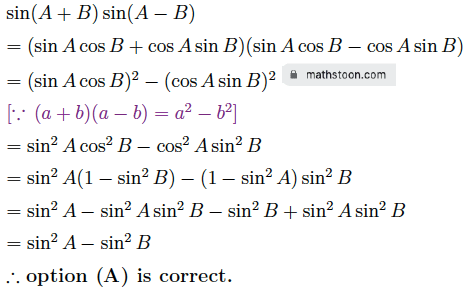Ex 2:  cos(A+B) cos(A-B)

(A) sin2A – sin2(B) cos2A – sin2B   (C) cos2B – sin2B    (D) cos2A – cos2B

Solution: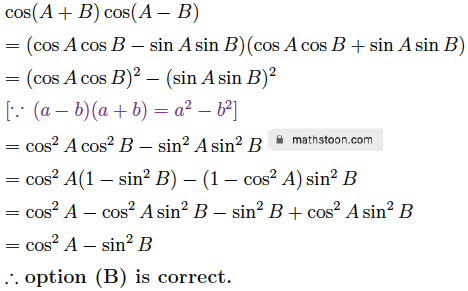Ex 3:  sin(45°-θ)

(A) $\dfrac{1}{\sqrt{3}}$(sinθ – cosθ)  (B) $\dfrac{1}{\sqrt{2}}$(sinθ – cosθ)   (C) $\dfrac{1}{\sqrt{3}}$(cosθ – sinθ)    (D) $\dfrac{1}{\sqrt{2}}$(cosθ – sinθ)

Solution:

sin(45°-θ)

= sin45° cosθ – cos45° sinθ

= $\dfrac{1}{\sqrt{2}}$ cosθ – $\dfrac{1}{\sqrt{2}}$ sinθ

= $\dfrac{1}{\sqrt{2}}$ (cosθ – sinθ)

∴ Option (D) is correct.

Ex 4:  tan(π/4+θ) tan(π/4-θ)

(A) 0  (B) $\dfrac{1}{\sqrt{3}}$   (C) 1   (D) $\sqrt{3}$

Solution: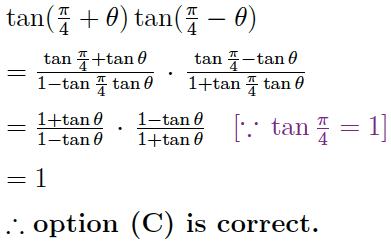Ex 5:  cot 2θ + tan θ =

(A) sin22θ  (B) cot22θ   (C) cosec22θ   (D) tan2

Solution: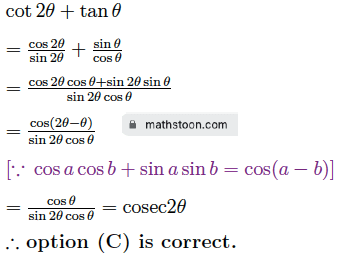Ex 6:  2cos(π/3+A) =

(A) cosA – √3sinA  (B) cosA – √2sinA   (C) sinA – √3cosA   (D) sinA – √2cosA

Solution: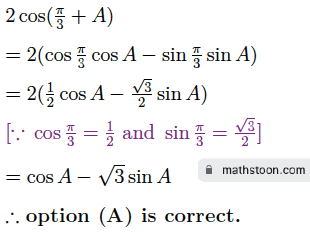Ex 7:  If sinA = $\dfrac{3}{5}$, cosB = $-\dfrac{12}{13}$, where A and B both lie in second quadrant, then the value of sin(A+B) is –

(A) $\dfrac{56}{65}$  (B) $-\dfrac{56}{65}$   (C) $\dfrac{65}{56}$   (D) $-\dfrac{65}{56}$

Solution:

As both A and B lie in second quadrant, the value of cosA and sinB are respectively negative and positive.

∴ cosA = $-\sqrt{1-\sin^2 A}$ $=-\sqrt{1-\dfrac{9}{25}}$ $=-\sqrt{\dfrac{16}{25}}$ $=-\dfrac{4}{5}$ and

sinB = $\sqrt{1-\cos^2 B}$ $=\sqrt{1-\dfrac{144}{169}}$ $=\sqrt{\dfrac{25}{169}}$ $=\dfrac{5}{13}$

Now, sin(A+B)

= sinA cosB + cosA sinB

= $\dfrac{3}{5} \cdot (-\dfrac{12}{13})$ + $(-\dfrac{4}{5}) \cdot \dfrac{5}{13}$

= $-\dfrac{36}{65}-\dfrac{20}{65}$

= $-\dfrac{36+20}{65}$

= $-\dfrac{56}{65}$

∴ Option (B) is correct.

Ex 8:  $\dfrac{\cos 9^\circ +\sin 9^\circ}{\cos 9^\circ -\sin 9^\circ}$

(A) sin54°  (B) cos54°   (C) tan54°   (D) cot54°

Solution: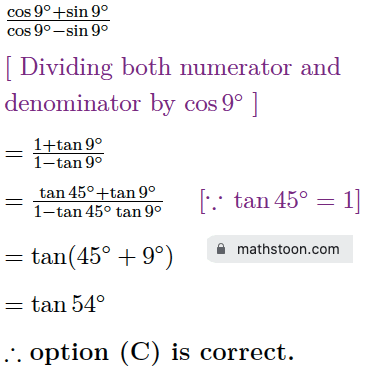Ex 9:  If sinA + sinB = 2, then which of the following is the value of sin(A+B)?

(A) 2  (B) 0   (C) 1   (D) -1

Solution: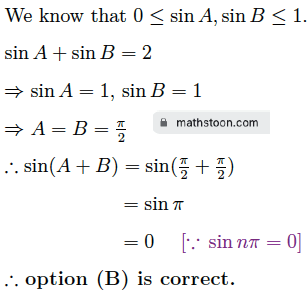Ex 10:  If sinθ + sinφ = 2, then which of the following is the value of cos(θ+φ)?

(A) 0  (B) 1   (C) -1   (D) 2

Solution: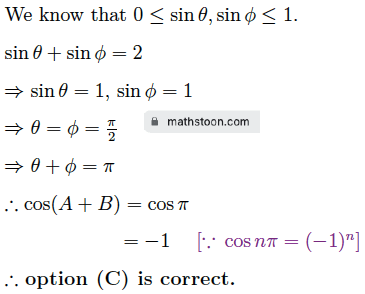Ex 11:  If cosA + cosB = 2, then which of the following is the value of cos(A+B)?

(A) 1  (B) 0   (C) -1   (D) 2

Solution:

As both cosA and cosB lie between 0 and 1, we have:

cosA + cosB = 2

⇒ cos A =1, cos B =1

⇒ A=0° and B=0°

⇒ A+B=0°

∴ cos(A+B) = cos 0° = 1.

∴ Option (A) is correct.

Ex 12:  If tanA = $\dfrac{3}{4}$ and tanB = $\dfrac{4}{3}$, then which of the following is the value of (A+B)?

(A) $\dfrac{\pi}{4}$  (B) $\dfrac{3\pi}{4}$   (C) $\pi$   (D) $\dfrac{\pi}{2}$

Solution: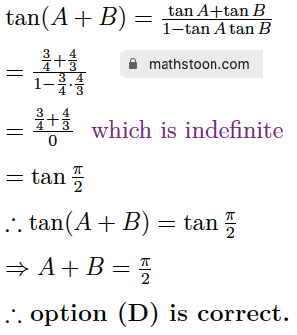Share via: